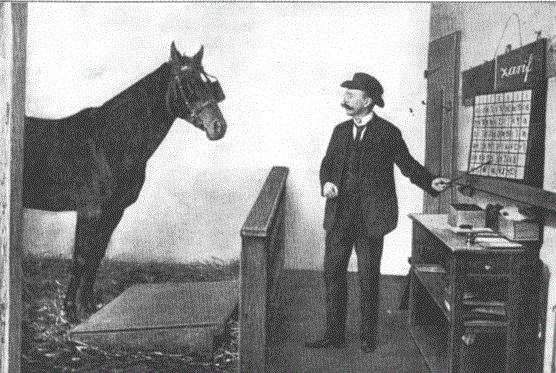# Evaluate Improper Integrals with Beta FunctionEarlier we’ve talked about gamma and beta functions.You’re probably interested, what beta function is useful for? In this section we’ll show how to integrate certain functions in simpler way with the help of beta function. Suppose we need to evaluate the following integral:

$$\int_0^{3}{x^{1/2}(27-x^3)^{-1/2} dx}$$

At first glance we can say that there is not an easy expression to deal with under the integral sign. As you can see we have radicals and powers under the integral sign so it’s not an easy thing to integrate directly. Moreover, take a look at the expression in braces: as it’s in negative power it means this is a denominator; and at the upper limit this denominator turns into zero. In other words, we’re facing an improper integral. And that’s what Euler’s functions are about! But which one should  we take – gamma or beta? Recall them both:

$$B(p,q)=\int_0^{1}{x^{p-1}(1-x)^{q-1}dx}$$

$$\Gamma(p)=\int_0^{\infty}{e^{-x}x^{p-1}dx}$$

Watch video tutorial on our youtube channel:The given integral has limits of integration $0,3$. Beta function has limits $0,1$. So let’s try to change given integral to obtain beta function. By the way, compare it with the case when gamma function works, an example we’ve considered earlier. First let’s factor $27$ out of the braces:

$$\int_0^{3}{x^{1/2} (27-x^3 )^{-1/2}dx}=\int_0^{3}{x^{1/2}27^{-1/2} (1-\frac{x^3}{27})^{-1/2}dx}$$

Now let’s make a substitution:

$$\frac{x}{3}=t \rightarrow x=3, dx=3dt$$Be careful with limits of integration which are also to be changed:

$$x=0 \rightarrow t=0$$

$$x=3 \rightarrow t=1$$

After making this substitution, we obtain:

$$\int_0^{3}{x^{1/2}27^{-1/2} (1-\frac{x^3}{27})^{-1/2}dx}=27^{-1/2} \int_0^{1}{3^{1/2} t^{1/2} (1-t^3 )^{-1/2}3dt}=\int_0^{1}{t^{1/2} (1-t^3 )^{-1/2}dt}$$

Now we have an integral with required limits $0,1$ but it’s still not beta function. You see $t^3$ in braces, however, in beta function there’s the first power of the argument. Let’s make another substitution to get rid of this dissimilarity:

$$t^3=u \rightarrow t=u^{1/3}, dt=\frac{1}{3}u^{-2/3}$$

Limits of integration remain the same (nevertheless, don’t forget to check this each time you make a substitution):

$$t=0 \rightarrow u=0$$

$$t=1 \rightarrow u=1$$

Therefore, we obtain:

\begin{aligned}\int_0^{1}{t^{1/2} (1-t^3 )^{-1/2}dt}=\int_0^{1}{u^{1/6} (1-u)^{-1/2} \frac{1}{3}u^{-2/3}du}=\frac{1}{3}\int_0^{1}{u^{-1/2} (1-u)^{-1/2}du}&\\=\frac{1}{3} B(\frac{1}{2},\frac{1}{2})\end{aligned}

Finally, we’ve got desired beta function. However, that’s not the answer yet. Now we can use formula relating beta and gamma functions and express obtained result through gamma functions (that’s because we know how to evaluate them). let’s recall it first:

$$B(p,q)=\frac{\Gamma(p)\Gamma(q)}{\Gamma(p+q)}$$

Substituting this into our expression we obtain:

$$\frac{1}{3}B(\frac{1}{2},\frac{1}{2})=\frac{1}{3}\cdot \frac{Γ(\frac{1}{2})Γ(\frac{1}{2})}{Γ(1)}=\frac{1}{3}\frac{\sqrt{\pi}\sqrt{\pi}} {1}=\frac{\pi}{3}$$

And that’s the answer. By the way, improper integrals (and ours is indeed such a one, as we found out above) which can be evaluated and yield finite number are usually called convergent. If you ever take an integral calculus class, you’ll know what this implies exactly and how to check it for this or that integrand. That’s all for now, don’t miss our next sections! Do math.0 Shares
Filed under Math.